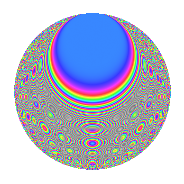# Properties

 Label 1008.2.zLevel 1008 Weight 2 Character orbit z Rep. character $$\chi_{1008}(253,\cdot)$$ Character field $$\Q(\zeta_{4})$$ Dimension 120 Sturm bound 384

# Related objects

## Defining parameters

 Level: $$N$$ $$=$$ $$1008 = 2^{4} \cdot 3^{2} \cdot 7$$ Weight: $$k$$ $$=$$ $$2$$ Character orbit: $$[\chi]$$ $$=$$ 1008.z (of order $$4$$ and degree $$2$$) Character conductor: $$\operatorname{cond}(\chi)$$ $$=$$ $$16$$ Character field: $$\Q(i)$$ Sturm bound: $$384$$

## Dimensions

The following table gives the dimensions of various subspaces of $$M_{2}(1008, [\chi])$$.

Total New Old
Modular forms 400 120 280
Cusp forms 368 120 248
Eisenstein series 32 0 32

## Trace form

 $$120q - 2q^{4} + O(q^{10})$$ $$120q - 2q^{4} - 8q^{10} - 4q^{11} - 2q^{14} + 10q^{16} + 16q^{19} + 28q^{20} - 14q^{22} + 52q^{26} - 8q^{29} + 28q^{34} - 8q^{37} - 56q^{38} + 20q^{40} + 36q^{43} - 26q^{44} - 48q^{46} - 120q^{49} - 86q^{50} - 16q^{52} + 8q^{53} + 14q^{56} + 34q^{58} + 24q^{59} - 32q^{61} + 12q^{62} - 50q^{64} - 16q^{65} + 4q^{67} + 104q^{68} - 24q^{70} + 22q^{74} + 80q^{76} + 8q^{77} - 72q^{79} + 68q^{80} + 44q^{82} - 40q^{83} + 32q^{85} - 2q^{86} - 94q^{88} - 100q^{92} - 52q^{94} - 64q^{95} + O(q^{100})$$

## Decomposition of $$S_{2}^{\mathrm{new}}(1008, [\chi])$$ into newform subspaces

The newforms in this space have not yet been added to the LMFDB.

## Decomposition of $$S_{2}^{\mathrm{old}}(1008, [\chi])$$ into lower level spaces

$$S_{2}^{\mathrm{old}}(1008, [\chi]) \cong$$ $$S_{2}^{\mathrm{new}}(16, [\chi])$$$$^{\oplus 6}$$$$\oplus$$$$S_{2}^{\mathrm{new}}(48, [\chi])$$$$^{\oplus 4}$$$$\oplus$$$$S_{2}^{\mathrm{new}}(112, [\chi])$$$$^{\oplus 3}$$$$\oplus$$$$S_{2}^{\mathrm{new}}(144, [\chi])$$$$^{\oplus 2}$$$$\oplus$$$$S_{2}^{\mathrm{new}}(336, [\chi])$$$$^{\oplus 2}$$

## Hecke characteristic polynomials

There are no characteristic polynomials of Hecke operators in the database